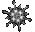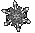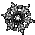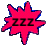Averages Game with Range. Arithmetic Mean. Median. Mode.    Math Help

- It may help, if you arrange the given set of numbers in order from smallest to largest.
You can rearrange a data set such as {30,40,20,10} to become {10,20,30,40}.

- The range of a set of data values is equal to the largest value minus the smallest value.
The range of {30,50,20,10} is found by doing the subtraction 50-10 to get the range = 40.

- The mean of a set of data values = the sum of the data values divided by the number of data values.
To find the mean of {25,25,40}, use addition 25+25+40=90 and the division 90/3 = mean = 30.

- The median = the middle value of an odd number of values arranged in order
(ascending or descending).
The median of the five values {10,25,35,50,50} is 35.

- For an even number of ordered values, the median is the mean of the two middle values.
The median of the four values {5,10,30,60} is (10+30)/2 = 20.

- The mode = the number value which occurs most frequently in a set of data values.
The mode of {50,25,35,40,25} is 25.

- Your Game Score is reduced by the number of snowflake hits.

- To slow the game speed repeat tap/click on the word Slider.
- To increase the game speed repeat tap/click on the word Math.
- Speed can also be adjusted with a keyboard's - and + keys.

- Refresh/Reload the web page to restart the game.